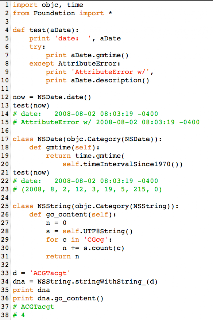## Monday, August 11, 2008

### PAM: Projecting in time

In the last post, we looked at the way Dayhoff et al. generated the PAM1 matrix. That matrix contains transition frequencies for changing one amino acid to another, based on observed changes in closely related proteins. The form of the matrix presented has all values multiplied by 10000 to make it easier to scan by eye. The total of each column sums to 10000, for a total probability of 1 that the amino acid will do something: either change or stay the same. According to the text:

The probability of observing a change in a site containing alanine (the sum of all the elements MXA except MAA) is proportional to the mutability of alanine. The same proportionality constant, lambda, holds for all columns. The individual nondiagonal terms within each column bear the same ratio to each other as do the observed mutations in the matrix of Figure 80.

The quantity 100 X the sum of fi Mii gives the number of amino acids that will remain unchanged when a protein 100 links long, of average composition, is exposed to the evolutionary change represented by this matrix. This apparent evolutionary change depends upon the choice of lambda, in this case, chosen so that this change is 1 mutation.

The paper goes on to discuss the use of PAM1 for simulating mutations.

The transition matrix PAM1 has the amino acid frequencies built into it. (Go back and see how it was computed). We can also see this by applying the matrix to a sequence (any sequence) many times. When you do that, the sequence approaches asymptotically the observed amino acid composition (frequencies).

We obtain the PAM matrix for longer times by matrix multiplication. To see the rationale for this, consider a period of two PAMs (twice the unit time to give 1 % mutation). If we observe D => E during this whole period, we can calculate the probabilities by observing that the position holding D at time-zero was something at PAM1, and then something turned into E by PAM2. For each possible something, X, we need to multiply the probability that D => X by the probability that X => E, and sum this over all possible X. We obtain the first probability by going to the column for D and scanning down to the row for X; we obtain the second probability by going to the row for E and scanning out to the column for X. The sum over all X is the entry for column D row E for PAM2.

This is exactly what matrix multiplication does. When we do matrix A x matrix B (or A %*% B in R), we take a particular row of matrix A corresponding to the amino acid E (the final result), and a particular column of B (correspnonding to the starting amino D) and take each element pairwise of the two and multiply them together (for each intermediate amino acid), then sum the result. To go from PAMn to PAMn+1 we multiply PAMn %*% PAM1. (Even though matrix multiplication is not commutative, it seems not to matter whether we do PAMn %*% PAM1 or PAM1 %*% PAMn repeatedly. I'm not sure why this is so, but I've tried the R code both ways and get the same result).

Substitution matrices are commonly used in log odds form. To get there we have to do two things: normalization (get the odds) and then take the log. The normalization consists of dividing by the amino acid frequency. That is, for each column of the PAM matrix, we have a set of frequencies with which the starting amino acid will turn into something else. We normalize each of those probabilities by dividing by the frequency of the new amino acid. (The probability that A => R is normalized to the frequency of R). And since the PAM1 entries are observed occurrences divided by fj, the odds matrix is just observed occurrences divided by fi * fj.

The base of the logarithm varies with the application, but in this paper they use base 10.

Here is a screenshot of my R code with a corrected form of PAM1, where I've proofread all the entries to be consistent with what's in the paper. There are still a few minor differences in the result, but it tracks pretty closely. As a final twist, the amino acids have been reordered to group those with like properties.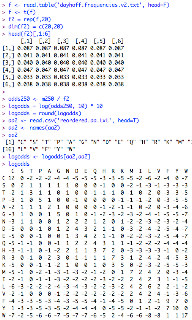### PAM: Point Accepted Mutation

I want to spend some time talking about substitution matrices and Markov models.These matrices underly most (all?) methods for protein sequence comparison. They also reflect an underlying model of a simple Markov chain which describes how each position in a sequence changes stochastically with time.

The first one that I know of is the PAM matrix, developed by Margaret Dayhoff and colleagues. The reference is the 1978 paper listed on the page above, but it isn't in PubMed. I see there is now a link to a pdf on the web. (It's behind a firewall now, sorry). I got it from my library, but my copy isn't any better than the one above, where some numbers in the first figure aren't legible. In fact, my copy looks like exactly the same crappy scan that is up.

If you are learning Bioinformatics (and I assume you are if you are reading this) I recommend that you take the time to read the paper carefully and reproduce the results. It's well worth the investment.

It's curious that the paper talks about "accepted point mutations" and PAM, but never explicitly makes the link or explains why they rearranged the order of the letters in the acronym.

They started with the catalogued proteins in the "Atlas of Protein Sequence and Structure" in 1978, and collected 34 sets of closely related "superfamilies" grouped into 71 evolutionary trees. Sequences within a tree were < 15% different. So these are quite closely related sequences. The method for making the trees was parsimony, and ancestral sequences were reconstructed. They give an example, shown here:Because B is present in the second position for both the second and the fourth sequences at the top (present day), it is inferred to be ancestral for the first and third. Each change on the tree was recorded in both directions. If they infer A => C, they record 1 for C => A as well. They indicate that because the trees could not always be completely determined, in such cases the substitution was apportioned among all possibilities according to the amino acid frequencies in the data. Because of this, they had fractional values, which were removed by multiplying the entire matrix by 10. Thus, the final version has 1572 changes, each counted twice, times 10 = 31440. I transcribed their first Figure, guessing at a few of the numbers, and my total is 31480. So I have four extra changes counted in my data set.

The next piece of data is the table of frequencies for the amino acids in their data set. This is called "Normalized Frequences...in the Accepted Point Mutation Data," which is a bit confusing because it is not related to the mutation data, just to the protein sequences the started with. They normalize the observed changes involving each amino acid to the frequency in the sequences (what they call its "exposure"). They call this value relative mutability, and it varies over nearly an order of magnitude. It reflects the entire process of evolution (i.e. rates of mutation and rates of acceptance during evolutionary time). Here is the table I generated from their data. The columns are amino acid, observed changes, amino acid frequency, and the last column is the relative mutability, scaled to alanine = 100%):In the last part of the first half, they calculate a new matrix based on the data. This is going to be the matrix of transmission frequencies for our Markov model. The matrix is arranged with "original" amino acids as the column heads, and "replacement" amino acids as the rows. (This is the opposite of the way it is normally done today). Each column in the matrix shows the probability that the indicated "original" amino acid will change to a different one, or stay the same. To make the table easy to look at, their formulation has integer values which sum to 10000. So you must divide by 10000 to get the actual frequencies. Their formula is:The subscript i refers to rows (new amino acid) and j refers to columns (old amino acid). The matrix entry is calculated as a constant (lambda) times the mutability for the old amino acid times the number of changes seen between old and new, divided by all changes seen for the old amino acid.

There is an interesting bit of circular reasoning in construction of the matrix. Both mutability and total changes appear in the formula, but mutability has already been calculated in terms of the sum of all changes for that amino acid. So an equivalent formulation is:where K is a different constant and f is frequency. I wrote a Python script to calculate the PAM1 matrix from their data. Here is the resulting matrix, loaded into R. It is nearly the same as theirs, but not identical, probably because of transcription errors in the first table.## Sunday, August 10, 2008

### GC content

Before I leave Ch 2 of Deonier behind, I have one last program to show. The goal is similar to previously, we want to look at GC-content in a window sliding across the genome. But we want to make the window smaller, and rather than look at the Chi-squared statistic, we'll just plot %GC. The first program I wrote generates this (when plotted in R):The statistics (number of intervals with GC-content in the indicated range) indicate many more low GC segments than expected. I have to check this, but I think that for a binomial distribution with p = 0.5, the standard deviation is the square root of n * 0.5 * 0.5 or 5 for 100 trials. So, we'd expect 99% of random trials to have GC > 35%. What we observe is that 1595 / 46397 are < 35%, about 3.4%.

 ` > 75 3< 25 123 > 70 32< 30 531> 65 347< 35 1595`

 `# window and step sizeW = 100results = list()s = seq[:W]seq = seq[W:]start = time.time()while s: results.append( str(s.count('G') + s.count('C'))) s = seq[:W] seq = seq[W:]print '%3.2f' % (time.time() - start)# 254.33`

However, as indicated by the comment, it takes an embarassingly long time to run---almost 4 minutes. This is the way I would normally step through a sequence but I got an idea to make it faster. That is to step through the sequence 1 nt at a time with two pointers separated by 100 nt. If the leading pointer hits G or C, we increment the count, and if the lagging pointer hits G or C we decrease it by one. We start with a GC content at the equilibrium value and save every 100 nt. Notably, it runs much faster than the other code, but the plot we generate is subtly different. Well actually, it's not that subtle---the average looks to be shifted up near 57% and the while the patterns are clearly related, they are not the same. I'm not sure why, at the moment.

 `# window and step sizeW = 100SZ = len(seq)results = list()print SZGC = 50start = time.time()for i in range(SZ-W): j = i + W nt = seq[i] if nt == 'G' or nt == 'C': GC -= 1 nt = seq[j] if nt == 'G' or nt == 'C': GC += 1 if not i % 100: results.append(str(GC)) print '%3.2f' % (time.time() - start)# 10.47`

### Deonier Ch 2 frequency bias

My last example for Ch 2 is inspired by exercise 11. We load the E. coli genome sequence, and determine the expected count of 'ACGT' per 1 kb, based on the overall nucleotide composition. Then we slide a window of 1 kb along the genome and compare the observed count to the expected, computing the chiSquared statistic like this:

 `def chiSquared(s, v=False): if len(s) != 1000: print 'error, wrong length' return total = 0 for c in b: o = s.count(c) e = eD[c] statistic = 1.0*(o-e)**2/e total += statistic if v: print c, o, e, if v: print '%3.2f' % statistic, if v: print '%3.2f' % total return total`

We plot the results using R. Notice that segments with high bias rise above the noise, and these segments tend to cluster, as marked by the vertical red line:If we take a closer look, we resolve particular segments involved.This region around 3796 - 3800 kb is involved in LPS biosynthesis. This is a screenshot from my GeneFinder app.Here is a closeup of Fig 1 in Lawrence and Ochman 1998.And here is part of an abstract of a paper noting the lack of conservation between E. coli and Salmonella. As far as I can tell, the functions of the the genes with very high bias aren't known, but presumably their lack of conservation is related to evolutionary changes in LPS.If we set the verbose flag, we can go back to this segment and look at the values:

 `3794 A 263 C 225 G 278 T 234 7.343795 A 326 C 162 G 149 T 363 158.393796 A 321 C 159 G 173 T 347 125.703797 A 345 C 158 G 151 T 346 158.543798 A 329 C 180 G 160 T 331 113.723799 A 332 C 181 G 153 T 334 122.693800 A 314 C 196 G 171 T 319 80.833801 A 310 C 210 G 180 T 300 57.693802 A 335 C 176 G 122 T 367 184.273803 A 282 C 220 G 175 T 323 58.493804 A 271 C 237 G 213 T 279 14.723805 A 276 C 247 G 205 T 272 16.053806 A 293 C 231 G 210 T 266 20.313807 A 217 C 273 G 266 T 244 5.42`

What would be nice at this point would be to filter the output for regions with extreme values and then recover and order all the ORFs in each. I'll leave that as an exercise for the reader. :)

### Deonier Ch 2 Codon Bias - Codon Data

Continuing with the problem of analyzing codon bias, there is abundant evidence that the preferred codon usage pattern varies greatly. There are several ideas about why this might be (see e.g. Andersson and Kurland 1990). What I'm concerned with here is to explore whether there is a relationship between expression level and codon usage in E. coli. Please note that this is not a scholarly examination of the question. I am really just trying to develop my scripting skills by reading and exploring Deonier.

In previous posts (1, 2) I discussed obtaining the data for all coding sequences (CDS) from the GenBank record for the genome of MG1655, and Affymetrix array expression data (for a rather distant relative, AB1157). Loading and sorting the data is straightforward. (See my script). I obtained expression data for 4345 genes. But only one of the top 50 in expression encodes a protein (lpp) We filter the expression data for CDS's (obtaining 2938 items). Here is a histogram of the values, scaled so that we can look at rare bins on the high end of the distribution.I chose genes with expression levels in the top 40 as representative of highly expressed genes (values to the right of the red vertical bar, > 276), and I chose genes with expression < 150 as representative of genes with average expression. Here are the top 12:

 `lpp 3343.85rmf 1953.51fimA 1112.27cspC 853.39yfiD 840.27rpmJ 759.78yiiU 635.30cspG 597.44hns 570.08cspE 549.95udp 537.76dnaK 470.56`

I also went back and looked at one of the original references (Sharp and Li, 1986, behind a firewall), which gives lists of very highly and highly expressed genes. Interestingly, only 5 of their very highest category qualify in the top 40.

From this point, it is simply a matter of counting all the codons used in each gene for each group and saving the counts in a dict. To analyze the results, for each amino acid, we compare the ratio of the count for a given codon to the total of the synonomous codons, and finally, we compute the ratio of ratios (high expression to average). Here are examples for two amino acids, serine and tyrosine, as well as stop codons:

 `S TCG 22 0.059 8457 0.156 0.382S TCA 21 0.057 6449 0.119 0.478S AGT 37 0.100 7923 0.146 0.685S AGC 82 0.222 15247 0.281 0.789S TCC 91 0.246 8282 0.153 1.612S TCT 117 0.316 7909 0.146 2.170Y TAT 75 0.362 15155 0.561 0.646Y TAC 132 0.638 11852 0.439 1.453* TGA 8 0.200 796 0.282 0.710* TAG 2 0.050 198 0.070 0.714* TAA 30 0.750 1832 0.648 1.157`

The third and fourth columns are the codon count and frequency for highly expressed genes, the fifth and six columns are the same for average genes, and the last column is the ratio of ratios. It's clear that some codons are disfavored for highly expressed genes. For the stop codons, there are not enough examples to say anything with confidence.

Here are some more, where we compare the most and least favored codon for a particular amino acid:

 `A GCT 250 0.337 14131 0.158 2.138A GCC 116 0.156 24186 0.270 0.580C TGC 37 0.627 6080 0.564 1.111C TGT 22 0.373 4693 0.436 0.856D GAC 234 0.505 18400 0.378 1.338D GAT 229 0.495 30307 0.622 0.795E GAA 408 0.730 37809 0.691 1.056E GAG 151 0.270 16878 0.309 0.875F TTC 163 0.639 16091 0.435 1.471F TTT 92 0.361 20942 0.565 0.638G GGT 300 0.503 23714 0.339 1.483G GGA 23 0.039 7113 0.102 0.379H CAC 80 0.552 9403 0.438 1.260H CAT 65 0.448 12073 0.562 0.797I ATC 275 0.608 24210 0.426 1.428I ATA 10 0.022 3708 0.065 0.339K AAA 384 0.814 31585 0.768 1.059K AAG 88 0.186 9525 0.232 0.805L CTG 437 0.714 51779 0.509 1.402L CTA 10 0.016 3588 0.035 0.463M ATG 184 1.000 26359 1.000 1.000N AAC 258 0.789 20608 0.560 1.409N AAT 69 0.211 16185 0.440 0.480P CCG 164 0.672 22815 0.542 1.240P CCC 7 0.029 4951 0.118 0.244Q CAG 272 0.791 27460 0.658 1.201Q CAA 72 0.209 14248 0.342 0.613R CGT 234 0.578 20306 0.390 1.480R CGG 11 0.027 4727 0.091 0.299S TCT 117 0.316 7909 0.146 2.170S TCG 22 0.059 8457 0.156 0.382T ACT 152 0.328 8277 0.164 2.001T ACG 57 0.123 13497 0.267 0.460V GTT 261 0.441 17052 0.254 1.735V GTC 66 0.111 14442 0.215 0.518`

### Deonier Ch 2 Codon Bias - Expression Data

Next, we want some expression data for E. coli genes. There are lots of sources for this, but I went to GEO, where I found the page shown in the screenshot. The study is described here.It seemed like a good set because of the growth protocol: "wild type" cells pre-grown in rich medium, starved in salts, and then diluted in rich medium and grown for 90 minutes. Here is the description:The cells are AB1157, which are quite a long way from their ancestral purity, but we'll ignore that:The data are available in several formats including "Series Matrix" which is basically text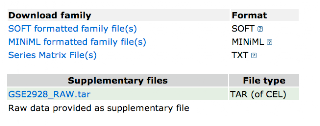It's a simple matter to process this to a format with recognizable gene names, and values for the measured expression levels.

### BioPython for GenBank

I was up in the dusty attic of my computer yesterday, looking through old code, and I found an example using BioPython to get the CDS entries from a GenBank record. It uses a Cocoa API to do the save, but that's all. It's probably preferable to the hand-rolled code from the last post.

 `from Bio import GenBankfrom Foundation import *from string import maketrans, upperFH = open('sequences.gb', 'r')parser = GenBank.FeatureParser()it = GenBank.Iterator(FH, parser)results = list()def processGene(f): D = dict() D['start'] = int(f.location._start.position) D['end'] = int(f.location._end.position) D['strand'] = int(f.strand) D['bid'] = f.qualifiers['locus_tag'] D['name'] = f.qualifiers['gene'] return Drecord = it.next()L = record.featuresL = [f for f in L if f.type == 'CDS']L = [processGene(f) for f in L]sequence = record.seq.tostring()print len(L)def reversesequence(s): t = maketrans('agctAGCT','tcgaTCGA') s2 = s.translate(t) L = list(s2) L.reverse() return ''.join(L)def getsequence(D): global sequence seq = sequence[D['start']:D['end']] if D['strand'] == -1: seq = reversesequence(seq) D['sequence'] = seq return D L = [getsequence(D) for D in L]filename = NSHomeDirectory() filename += '/Desktop/ECgenes.plist'ma = NSArray.arrayWithArray_(L)ma.writeToFile_atomically_(filename, True)`

## Saturday, August 9, 2008

### Deonier Ch 2 Codon Adaptation Index (1)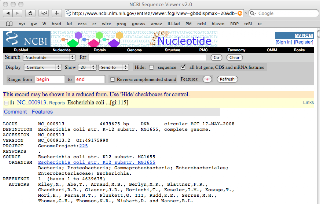The last part of Ch. 2 talks about k-words with k > 2, e.g. codons. About 20 years ago people first noticed that the codons used by highly expressed genes seemed to be a subset of possible ones. I'll discuss why this should be in a later post, and I'll also postpone discussion of a test of the hypothesis

But that is where we are headed, using the genome sequence and array data on expression levels. The first thing is to get the sequences of all the E. coli genes. Rather than look around for this resource or use BioPython I decided to do it (quickly I thought) by hand. The difficulty is that this code is always finicky to write. I went to the page listing bacterial genomes at NCBI and grabbed the genome sequence (NC_000913) in text format, making sure to include the DNA sequence. Then I just ran the script below, which parses all CDS entries, obtains the gene name and sequence, and writes the result to disk. We filter out two complex genes (multiple ORFs), and throw away 85 entries which seem to be mis-annotated. The result contains 4157 gene sequences, which we save in a text file.

 `# file contains NC_000913 as GenBank format# has the sequenceimport string, sysdata = open('EC.genome.txt','r').read().strip()locus, rest = data.split('\n',1)rest, seq = rest.split('ORIGIN')seq = ''.join([c for c in seq if c in 'acgt'])print locus[:50]print len(seq)#---------------------------------------------def process(L): def getName(s): # e.g. '/gene="ileV"' return s.split('=').replace('"','') def getCoord(s): # e.g. 'complement(287628..288386)' if '(' in s: s = s.split('(',1).replace(')','') stop,start = s.split('..') else: start,stop = s.split('..') return start,stop # multiple segments if L.count('..') > 1: return first, second = L,L first = first.split().strip() start,stop = getCoord(first) g = getName(second.strip()) # global CDSList.append( (g, start, stop) )# we use CDS b/c we know they are proteins# we want the CDS coordinates (on same line)# but we also want the CDS name# usually on the next line but not always!def getCDSList(s): lines = s.split('\n') line = lines.pop(0) D = dict() while line: if CDS in line: L = [line] L.append(lines.pop(0)) if gene in L[-1]: process(L) else: # split gene pass line = lines.pop(0)#---------------------------------------------def reversecomplement(s): tt = string.maketrans('ACGTacgt','TGCAtgca') s = string.translate(s,tt) L = list(s) L.reverse() return ''.join(L) def groupSequence(seq,SZ=100): L = list() s = seq[:SZ] seq = seq[SZ:] while s: L.append(s) s = seq[:SZ] seq = seq[SZ:] return '\n'.join(L)#---------------------------------------------CDS = ' CDS 'gene = '/gene'CDSList = list()getCDSList(rest)results = list()def show(g,s): print g,s[:12] + '..' + s[-12:]for t in CDSList: g, start, stop = t start = int(start) stop = int(stop) if start < stop: s = seq[start-1:stop] else: s = seq[stop-1:start] s = reversecomplement(s) s = s.upper() # 85 are apparently mis-annotated # 29 of them have names e.g. pcnB if not s[:3] in ['ATG','GTG','TTG']\ or not s[-3:] in ['TAG','TGA','TAA']: if not g == 'y': show(g,s) else: s = groupSequence(s) results.append('>' + g +'\n' + s) print len(results)FH = open('EC.genes.txt','w')FH.write('\n\n'.join(results))FH.close()`

### Python version of simple Markov chain

Here is Python code to generate a simple Markov chain. The first part is what we were given for the Deonier example. The second part is mainly a utility function to simulate what R's "sample" does, choose an item randomly from a sequence, based on a frequency distribution. The third part constructs the model and tests it.
 `import random,sysnt = 'ACGT'dinuc = [n1 + n2 for n1 in nt for n2 in nt]R = random.Random(1531)# what do we need for a Markov process?# example: dinucleotide frequencies# start with a list of dinucleotide frequencies# nt order is ACGT, row 1..4 are A..T as 1st nt# cols 1..4 are A..T as 2nd ntdef initDiNucleotideDict(): L = [ 0.146, 0.052, 0.058, 0.089, 0.063, 0.029, 0.010, 0.056, 0.050, 0.030, 0.028, 0.051, 0.087, 0.047, 0.063, 0.140 ] # organize L into a dictionary of dinucleotides return dict(zip(dinuc,L)) #------------------------------------------------- # compute individual nt probabilities pNdef initMonoNucleotideDict(nnD): D = dict(zip(list(nt), *len(nt))) for nn in dinuc: n = nn D[n] += nnD[nn] return D #------------------------------------------------- # P(nn) is the observed dinucleotide freq in nnD# P(nn) = P(n1) * P for transition from n1 to n2# i.e. P(n1 => n2) = P(n1+n2) / P(n1)# since we will choose given n1# we need a dict of dictsdef initTransitionDict(nD,nnD): bigD = dict() for n1 in nt: D = dict() for n2 in nt: D[n2] = nnD[n1+n2] / nD[n1] bigD[n1] = D return bigD#-------------------------------------------------# utility function: randomly choose one item# from a list of tuples of (item,freq)# not necessarily summing to 1# allow a dict argumentdef randomChoice(L): if type(L) == type({}): L = L.items()[:] floor = 0 ceiling = sum([t for t in L]) f = R.random() while f > ceiling: f = R.random() for t in L: floor += t if f < floor: return tdef testRandomChoice(L): R = range(int(1E5)) L2 = [randomChoice(L) for i in R] for n in nt: print n, L2.count(n) def doInits(): nnD = initDiNucleotideDict() nD = initMonoNucleotideDict(nnD) tD = initTransitionDict(nD,nnD) return nD,nnD,tDdef testAll(): nD,nnD,tD = doInits() for n in nt: print n,'%3.3f' % nD[n] print testRandomChoice(nD.items()) print for nn in dinuc: print nn,'%3.4f' % nnD[nn] print for n1 in nt: for n2 in nt: print n1+n2,'%3.4f' % tD[n1][n2] # testAll(); sys.exit()#-------------------------------------------------def markov(nD,tD,SZ): L = [randomChoice(nD.items())] for i in range(SZ-1): D = tD[L[-1]] L.append(randomChoice(D.items())) return ''.join(L) def testmarkov(seq,nnD): D = dict(zip(dinuc, *len(dinuc))) SZ = len(seq) for i in range(1,SZ): D[seq[i-1:i+1]] += 1 for k in nnD.keys(): print k.ljust(4), '%3.4f' % nnD[k], '\t', print '%3.4f' % (D[k] * 1.0 / SZ)nD,nnD,tD = doInits()L = markov(nD,tD,int(1E5))print L[:30] + '\n'testmarkov(L,nnD)'''prints:TAGTTTGTTTACAATGTCATTACAGATGTTAA 0.1460 0.1458AC 0.0520 0.0512GT 0.0510 0.0518AG 0.0580 0.0576CC 0.0290 0.0290CA 0.0630 0.0624CG 0.0100 0.0095TT 0.1400 0.1444GG 0.0280 0.0276GC 0.0300 0.0303AT 0.0890 0.0888GA 0.0500 0.0484TG 0.0630 0.0635TA 0.0870 0.0867TC 0.0470 0.0467CT 0.0560 0.0563'''`

### Elementary Markov Chain

In this section (Deonier Ch. 2 example 3) the Markov chain is introduced using the example of dinucleotide frequencies. (Here is Markov himself). In scanning a genome we might ask, given that the nucleotide at a certain position is 'A', what is the probability that the next nucleotide in the sequence is 'G'? We make the important (and obviously unrealistic) assumption that the next base depends only on the identity of the current one.

The Markov chain model we'll construct can be used to simulate DNA sequences having the properties built into the model. Now, imagine a genome not as a string of nucleotides, but consisting of individual positions that change through time. Each nucleotide in the sequence (or each amino acid residue in a polypeptide) can be though of as generated by a Markov chain through time. As far as I know, Margaret Dayhoff invented this idea. It is pervasive in phylogenetic analysis of sequences.

We have information in the form of a table of genomic dinucleotide frequencies. From this we can calculate the mononucleotide frequencies: freq('A') = sum(freq('AN')). The transition frequency is the probability of finding a particular base at position i+1, knowing the base at position i.

 `P(Xi is 'A' and Xi+1 is 'G') = P(Xi is 'A') * P(Xi+1 is 'G' | Xi is 'A')`

where the | means "given" (see Bayes rule). (And here is Bayes himself). The latter term is the transition probability. To calculate it, then, we divide the dinucleotide frequency by frequency of the first base.

Deonier have actually pre-computed the transition frequencies for M. genitalium, which makes their R program quite a bit shorter than my Python version. It uses the "sample" function of R, which helpfully takes a probability distribution as one of the arguments. Here is a screenshot of my transcription of the code executed in R: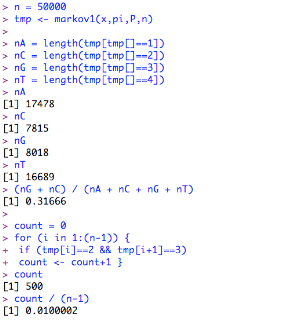## Friday, August 8, 2008

### Over-represented long oligonucleotides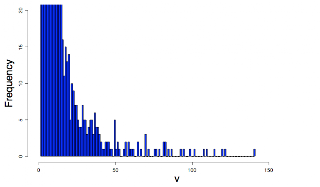We can look at the frequencies of longer oligos in the genome using Python. In this example, I look at the genome of Haemophilus influenzae because I know there is something interesting. The sequence is from Genbank L42023. On the average, we'd expect an individual 12-mer oligo (in a 50% GC genome) to be present once in 1.7 Mbp (4**12).

 `seq = open('Hinf.genome.txt','r').read().strip()title, seq = seq.split('\n',1)print titleseq = ''.join(seq.split())SZ = 12D = dict()for i in range(len(seq) - SZ): o = seq[i:i+SZ] if D.has_key(o): D[o] += 1 else: D[o] = 1def f(k): return D[k] for k in sorted(D.keys(),key=f)[-20:]: print k, D[k] FH = open('results.txt','w')FH.write('\n'.join([str(D[k]) for k in D.keys()]))`

It prints a list of oligos which are all related sequences except for the first one.
 `>gi|6626252|gb|L42023.1| Haemophilus influenzae Rd KW20, complete genomeTTGGTTGGTTGG 72AAAAGTGCGGTA 76TTAACCGCACTT 77TAAAAGTGCGGT 79AAAAGTGCGGTC 82TTTACCGCACTT 82AAAGTGCGGTAA 83ACCGCACTTTTA 83AAAAGTGCGGTT 85TTGACCGCACTT 87AAGTGCGGTTAA 93AACCGCACTTTT 95TTACCGCACTTT 99AAAGTGCGGTTA 102TAACCGCACTTT 108TGACCGCACTTT 110AAGTGCGGTCAA 115AAAAAGTGCGGT 120ACCGCACTTTTT 122AAAGTGCGGTCA 141`

We plot the results using R
 `setwd('Desktop')v = read.table('results.txt',head=F)[,1]mean(v)median(v)par('mar' = par('mar')+2)par(cex.lab = 2)hist(v,col='blue',breaks = 150, xlim=c(0,180),ylim=c(0,20),cex.lab=2)`

### Deonier Ch 2 simulation for example 2

Suppose we simulate a sequence of 1000 nt and count the frequency of one of the nucleotides, say 'A.' If the frequencies are all the same ( P(A) = 0.25 ), then we expect the mean count of a number of different simulations to be 250. Suppose we are looking at windows along a genome with an overall freq of P(A) = 0.25 and we observe a count of 280. What does that mean? Well, actually, it's a complicated situation because we would be asking the question many times. But suppose we just asked once, for one sequence, is 280 significantly different than 250? How do we decide?

One way is to do a simulation. We generate a random sequence big enough to sample 1000 times at 1000 nt each.
 `import random, time# random sequences of length = 1000# do this 10000 times and count 'A' each timelength = 1000trials = 1000start = time.time()seq = list('ACGT') * length * trialsrandom.shuffle(seq)middle = time.time()`
Now we run the simulations and count how many times there are more than 280 'A' nucleotides present:
 `results = list()for n in range(trials): i = n * length j = i + length results.append(seq[i:j].count('A'))T = 280 L = [n for n in results if n > T]print len(L) * 1.0 / trials# 0.014print '%3.2f seconds' % (middle - start)# 5.51 secondsprint '%3.2f seconds' % (time.time() - middle)# 0.06 seconds`

We'd only expect to obtain this result by chance 14 times in 1000.

### Dinucleotide frequencies

On p. 48-50 Deonier outline an analysis of dinucleotide frequencies. They use (what I think is) a modification of the Chi-square statistic, where the standard square of (O - E) divided by E is also divided by a normalizing constant they call c. This is some kind of correction for the fact that nucleotide frequencies aren't equal. I'm not going to post my code here, but I'll put a copy of the file up on the .mac server and it'll last as long as I renew my subscription. I really wish there was a way to post text files to Blogger. The first 1000 bp of genomic sequence for E. coli and M. genitalium were obtained from Genbank (NC_000913 and L43967). What took me more time than anything was to re-discover that string's count method is not suitable for this sort of thing. That's because of this behavior:
 `>>> s = 'GAAATTC'>>> s.count('AA')1>>> s = 'GAAAAAAAAAAAAAAAAAAAAAATTC'>>> s.count('AA')11`

This is what I get using the nucleotide frequencies assumed by Deonier. The first block is for E. coli. The second is for M. genitalium.

The columns are: dinucleotide, observed count, expected count, Chi-square, c, Chi-square/c. Note that my values are close but not quite the same as in the book. Also there is (not shown) quite a bit of difference when the true nucleotide frequencies are used, even though they aren't very different than those assumed for this analysis. The values in the last column > 1 indicate a discrepancy between the value expected based on single nucleotide frequency and that observed in the sequence.
 `AA 86 62.5000 8.8360 1.3125 6.7322AC 64 62.5000 0.0360 0.8125 0.0443AG 45 62.5000 4.9000 0.8125 6.0308AT 63 62.5000 0.0040 0.8125 0.0049CA 74 62.5000 2.1160 0.8125 2.6043CC 64 62.5000 0.0360 1.3125 0.0274CG 69 62.5000 0.6760 0.8125 0.8320CT 47 62.5000 3.8440 0.8125 4.7311GA 52 62.5000 1.7640 0.8125 2.1711GC 85 62.5000 8.1000 0.8125 9.9692GG 63 62.5000 0.0040 1.3125 0.0030GT 53 62.5000 1.4440 0.8125 1.7772TA 45 62.5000 4.9000 0.8125 6.0308TC 41 62.5000 7.3960 0.8125 9.1028TG 76 62.5000 2.9160 0.8125 3.5889TT 72 62.5000 1.4440 1.3125 1.1002AA 197 202.5000 0.1494 1.2925 0.1156AC 47 40.5000 1.0432 0.8785 1.1875AG 43 40.5000 0.1543 0.8785 0.1757AT 167 166.5000 0.0015 0.5005 0.0030CA 42 40.5000 0.0556 0.8785 0.0632CC 10 8.1000 0.4457 1.1557 0.3856CG 2 8.1000 4.5938 0.9757 4.7082CT 39 33.3000 0.9757 0.9001 1.0840GA 37 40.5000 0.3025 0.8785 0.3443GC 11 8.1000 1.0383 0.9757 1.0641GG 7 8.1000 0.1494 1.1557 0.1293GT 29 33.3000 0.5553 0.9001 0.6169TA 179 166.5000 0.9384 0.5005 1.8750TC 25 33.3000 2.0688 0.9001 2.2984TG 32 33.3000 0.0508 0.9001 0.0564TT 132 136.9000 0.1754 1.3293 0.1319`

### Deonier Ch 2 example 1,2

In example 2.1, Deonier want to simulate a DNA sequence by generating a random sequence of letters with some probability. Here is their code for a demo of the sample function in R:
 `x = c(1,0)pi = c(0.25,0.75)v <- sample(x,10,replace=T,pi)v#  1 0 0 0 1 0 0 0 1 0`

In Python I just make a list or string with the elements inserted according to the desired probability, then use random.choice.
 `import random, mathR = random.Random(31)x = [1,0,0,0]for i in range(20): print R.choice(x),print# 1 1 0 0 1 1 1 0 1 0 0 1 0 0 0 0 1 1 0 0`

To simulate a DNA sequence, they use the integers 1 to 4, because R doesn't deal with strings or characters very gracefully.
 `set.seed(13)pi = rep(0.25,4)x = 1:4seq1 <- sample(x,10000,replace=T,pi)seq1[1:15]#  4 2 3 2 1 2 4 1 1 2 4 1 1 4 4seq2 <- sample(x,10000,replace=T,pi)seq2[1:15]#  1 4 4 2 1 3 4 1 3 2 3 2 3 2 1`

In Python
 `b = 'ACGT'N = int(1E3)L = [R.choice(b) for i in range(N)]print L[:6]# ['A', 'C', 'C', 'T', 'A', 'A']print L.count('T')# 259`

In example 2.2, they generate a sequence from the binomial distribution
 `x <- rbinom(2000,1000,0.25)mean(x)#  250.0715var(x)#  196.1775sd(x)^2#  196.1775`

The math module doesn't have a mean function so we define some simple statistical functions:
 `def mean(L): return sum(L)*1.0/len(L)def var(L): m = mean(L) sumsq = sum([(n-m)**2 for n in L]) return 1.0/(len(L)-1) * sumsqdef sd(L): return math.sqrt(var(L))`

We fake the binomial distribution like this:
 `import random, mathR = random.Random(31)N = int(1E3)b = 'ACGT'x = list()obs = 2000for i in range(obs): L = [R.choice(b) for i in range(N)] x.append(L.count('T'))print '%3.2f %3.2f %3.2f' % ( mean(x), var(x), sd(x)) # 250.03 187.47 13.69`

And finally, we'd like to generate a histogram of the values (I'll plot the R results):
 `b = max(x) - min(x) + 1r = c(min(x)-10,max(x)+10)par('mar' = par('mar')+2)hist(x,breaks=b,xlim=r,xlab='successes', cex.lab=1.5,main='',col='magenta')`

### import random

I'm starting to work on Deonier again. I read about half of the book last summer and did some of the problems, but I need to revisit it. But first, a post about random numbers and choosing random elements from a sequence in Python. We use this a lot in simulations for Bioinformatics and in generating statistical confidence intervals.

Python has a module called "random" for generating such numbers. As the docs say:
Almost all module functions depend on the basic function random(), which generates a random float uniformly in the semi-open range [0.0, 1.0). Python uses the Mersenne Twister as the core generator. It produces 53-bit precision floats and has a period of 2**19937-1. The underlying implementation in C is both fast and threadsafe. The Mersenne Twister is one of the most extensively tested random number generators in existence.

Sometimes I've used an instance of the class random.Random(), but really this shouldn't be necessary unless you need random number generators that don't share state. If you seed the random number generator with the same integer seed, you'll get the same sequence. You can also seed with time.time(), which returns a float (in fact, this is the default if no seed is provided).

 `import randomR1 = random.Random(157)b = 'ACGT'L = [R1.choice(b) for i in range(20)]print ''.join(L)# GAACATAAAAGTCCCGACATR2 = random.Random(157)L2 = [R2.choice(b) for i in range(20)]print ''.join(L2)# GAACATAAAAGTCCCGACAT `

I use the functions "shuffle", "choice", and "sample" a lot. According to the help on my version of Python

 `Python 2.5.1 (r251:54863, Jan 17 2008, 19:35:17) [GCC 4.0.1 (Apple Inc. build 5465)] on darwin`

"sample" is supposed to leave the original population unchanged. I expected that it would be suitable for sampling with replacement. But, asking for more elements than exist in the population raises

 `ValueError: sample larger than population`

and the online docs are explicit that this function does sampling without replacement. Here is a recipe that does sampling with replacement suitable for very large sequences:

There are also various functions to allow sampling from distributions, e.g. "gauss" samples come from the normal distribution. In Chapter 2 Deonier wants to sample from the binomial distribution, which in R you do like this:

 `x <- rbinom(observations,trials,probability)`

Each observation consists of a number of trials with the stated probability of success for each trial, and what is reported is the number of successes in the observation.

 `t = 5o = 20p = 0.5v <- rbinom(o,t,p)v 2 2 0 2 2 2 2 3 3 4 3 3 0 4 3 3 1 2 1 2`

The Python random module does not provide a function to do this directly. What we can do is make a list which contains elements corresponding to success and failure with the correct ratio. Then we simple choose randomly from those with replacement, by using random.choice.

 `import random# suppose p = 0.25L = 'SFFF'# trials per observationR = range(5)result = list()for o in range(20): result.append( [random.choice(L) for t in R]) v = [r.count('S') for r in result]for n in v: print n,print`

 `# 1 2 0 1 1 2 2 1 3 0 2 1 0 2 1 2 0 2 1 0`

### NSToolbarToolbars add a nice professional look to an application. Shown above is a screenshot from a simple demo of toolbars using PyObjC. The toolbar's delegate (MyToolbarDelegate) implements the three required methods. In this example, we add a custom toolbar item called "Analyze":

 ` def toolbar_itemForItemIdentifier_willBeInsertedIntoToolbar_( self, toolbar, identifier, flag): if identifier == "Analyze": item = NSToolbarItem.alloc().initWithItemIdentifier_( "Analyze") item.setLabel_("Analyze") item.setPaletteLabel_("Analyze") item.setToolTip_("Analyze Your Gene") item.setImage_(NSImage.imageNamed_("GeneFinderIcon")) item.setTarget_(self.AD) sel = 'analyze:' item.setAction_(sel) return item return None def toolbarDefaultItemIdentifiers_(self, toolbar): return [NSToolbarCustomizeToolbarItemIdentifier, NSToolbarShowColorsItemIdentifier, "Analyze"] def toolbarAllowedItemIdentifiers_(self, toolbar): return [NSToolbarCustomizeToolbarItemIdentifier, NSToolbarShowColorsItemIdentifier, "Analyze", NSToolbarPrintItemIdentifier, NSToolbarShowFontsItemIdentifier, NSToolbarSpaceItemIdentifier, NSToolbarSeparatorItemIdentifier ]`

There is an icon added to the project in Resources.

We also need to initialize the toolbar and attach it to the window. I do this in a separate class called MyToolbarController. (All the outlets are set up as you'd expect, in the xib file). This one is relayed through the AppDelegate (AD).

 ` def awakeFromNib(self): self.toolbar = NSToolbar.alloc().initWithIdentifier_("toolbar") self.toolbar.setDelegate_(self.AD.myToolbarDelegate) print "delegate", self.toolbar.delegate() self.toolbar.insertItemWithItemIdentifier_atIndex_("Analyze", 0) for item in self.toolbar.items(): print item.itemIdentifier() self.toolbar.setDisplayMode_(NSToolbarDisplayModeIconOnly) # this allows customization as you'd expect self.toolbar.setAllowsUserCustomization_(True) self.toolbar.setAutosavesConfiguration_(True) self.myWindow.setToolbar_(self.toolbar) print "visible?", self.toolbar.isVisible()`

## Thursday, August 7, 2008

My initial attempts to include a C program file in a Cocoa-Python XCode project haven't worked. However, I do have a working example of using NSTask to run a C program as a separate process. I don't know if there is any advantage to doing it the Cocoa way (as opposed to using subprocess.Popen). The code in the AppDelegate is:

 `class PyXAppDelegate(NSObject): def applicationDidFinishLaunching_(self, sender): NSLog("Application did finish launching.") argL = ['13', '47', '36'] #p = NSHomeDirectory() + '/Desktop/example' p = NSBundle.mainBundle().pathForResource_ofType_('example', None) #print 'path', p t = NSTask.alloc().init() t.setLaunchPath_(p) t.setArguments_(argL) cent = NSNotificationCenter.defaultCenter() cent.addObserver_selector_name_object_( self, "checkATaskStatus:", NSTaskDidTerminateNotification, None) t.launch() @objc.signature('v@:@') def checkATaskStatus_(self, notification): status = notification.object().terminationStatus() if status == 0: print 'success' else: print 'error'`

The C file looks (amost) like this:

 `#include < stdio.h>#include < stdlib.h>#include < string.h>#include < math.h>int isPrime(int x) { int i; float limit = sqrt(x) + 1; for (i = 2; i < limit; i++) { if (!(x % i)) return 0; } return 1;}int main(int argc, char *argv[]){ printf("number of args = %i \n", argc); printf ("path %s\n", argv); int i; for (i = 1; i < argc; i++) { printf("arg #%i, ", i); int x = atoi(argv[i]); printf("x = %i, ", x); printf("isPrime = %i\n", isPrime(x)); } return 0;}`

It's compiled in the usual way

 `tom-elliotts-mac-mini-2:~ telliott_admin\$ gcc example.c -o example`

and then can be placed in a convenient directory, or more flexibly, added to the project. Notable, pathForResource_ofType_ will take 'None' for the second argument.

This is what it prints to the Console:

 `2008-08-07 19:44:05.325 PyX[3207:10b] Application did finish launching.number of args = 4 path /Users/telliott_admin/Cocoa.PyObjC/PyX/build/Release/PyX.app/Contents/Resources/examplearg #1, x = 13, isPrime = 1arg #2, x = 47, isPrime = 1arg #3, x = 36, isPrime = 0success`

### Python for simulations (3) - population structure

In an environmental sequencing project one isolates and sequences SSU (16S in bacteria) ribosomal RNA genes to obtain information about a microbial population. As you'd expect, the amount of detail you can see depends on the structure of the population and how hard you look. The goal is to estimate the total number of species (or phylotypes, for uncultivatable organisms), as well as their relative numbers. The problem is that the number of observations may be limited, although that is changing rapidly. Sophisticated methods (like DOTUR) exist to tackle this challenge, but I wanted to explore it in a simple way by using a simulation written in Python.

In the simulation, a population contains three frequency classes of species: high, medium and low. We construct a population with varying frequencies specified for the high and medium classes. For example, starting with a unit population size of 1000, we might specify a frequency for high of 0.50 (500 individuals, arranged in 10 species), a frequency for medium of 0.30 (300 individuals, arranged in 50 species), and the balance consisting of unique individuals (200 species).

Then, we sample from the population at random, with replacement. We make 1000 observations, and at each step we record the number of different species seen so far. To make this more reliable, we do five replicates and average the results. The code is simple enough that I'm not going to post it. If you would like a copy, shoot me an email. Here are the results, plotted using R:In the figure, each curve shows the number of species seen so far, as a function of the number of observations. The curves are labeled with the values for the high and medium frequency classes, as well as the total number of species actually present. As expected, the curves level off with increasing number of observations. But the rate at which this occurs depends greatly on the fraction of the population in the low frequency category. Also, it takes a lot of observations to get a reliable estimate of the total counts, I'd say about 10 times as many observations as there are species in all. Typical observation sets have 100-200 sequences where the estimated species count is between 100-1000.

## Tuesday, August 5, 2008

### PyObjC calls Objective-C names

your projects can be a mix of Objective-C, C, and C++ code
so I worked up an example. It is simpler than my previous one which depends on having a bundle already compiled.

The project is a Cocoa-Python application. The AppDelegate instantiates the class and calls the code we are going to write in Objective-C:

 ` def applicationDidFinishLaunching_(self, sender): NSLog("Application did finish launching.") e = example.alloc().init() NSLog("%s" % example.description()) s = e.fetchResource() print self.description(), s print c = e.fetchObject() print self.description() print c.description() n = e.compute_(10) print n`

The .h and .c files for the Objective C class that we've added to this project are here (some lines in this post are too long for the code window, copy and paste to a text file if you need to see it all). We've added a text file called "stuff.txt" to the project:

 `#import < Cocoa/Cocoa.h>@interface example : NSObject { NSColorList *CL; NSColor *c; int n;}- (NSString *)fetchResource;- (NSColor *)fetchObject;- (int)compute:(int) anInt; @end#import "example.h"@implementation example- (NSString *)fetchResource{ NSLog(@"%@", [self className]); NSString *path = [[NSBundle mainBundle] pathForResource:@"stuff" ofType:@"txt"]; NSLog(@"%@", [path description]); const char * cstring = [path cStringUsingEncoding:NSASCIIStringEncoding]; printf("path %s\n", cstring); NSString *s = [NSString stringWithCString:cstring]; return s; }- (NSColor *)fetchObject{ CL = [NSColorList colorListNamed:@"Crayons"]; c = [CL colorWithKey:@"Salmon"]; NSLog(@"%@", [c description]); //[c retain]; return c;}- (int)compute:(int) anInt{ n = anInt * 2; return n;}@end`

We can't import the example class from Python, we have to do this in "main.m." (There is an extra space in the import statement before I escape the <. I'm not that handy with html):

 `#import < Python/Python.h>#import < Cocoa/Cocoa.h>#import < example.h>`

And this is what it prints to the Console:

 `[Session started at 2008-08-05 19:07:39 -0400.]2008-08-05 19:07:40.872 PyBundle[5274:10b] Application did finish launching.2008-08-05 19:07:40.874 PyBundle[5274:10b] example2008-08-05 19:07:40.874 PyBundle[5274:10b] example2008-08-05 19:07:40.875 PyBundle[5274:10b] /Users/telliott_admin/Desktop/PyBundle/build/Release/PyBundle.app/Contents/Resources/stuff.txtpath /Users/telliott_admin/Desktop/PyBundle/build/Release/PyBundle.app/Contents/Resources/stuff.txt /Users/telliott_admin/Desktop/PyBundle/build/Release/PyBundle.app/Contents/Resources/stuff.txt2008-08-05 19:07:40.941 PyBundle[5274:10b] NSCalibratedRGBColorSpace 1 0.4 0.4 1NSCalibratedRGBColorSpace 1 0.4 0.4 120`

### Cocoa Heat Mapper

I started working on a Cocoa app that draws heat maps. It is pretty rough still, but works well enough to show a screenshot. Drawing the colored rectangles is entry-level NSView stuff. I added the ability to show the actual data in the squares. Since my data consists of counts of objects between 1 and 100 or so, this works fine. We switch the text color based on the background of the rectangle.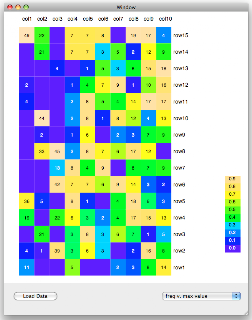In its current state it allows toggling between two different methods for assigning the colors. The first is to compute the frequency by dividing the count by the maximum value in that column, and the second just bases the assignment on the count. Adding additional methods is simple. I'm hoping to explore various methods of "turning up the contrast" to vary the level of visible detail in the data.

I didn't use bindings, except that the value of the popup is bound to a list of available methods, but the popup itself is wired up to the view like so:

 ` @objc.IBAction def popupChanged_(self, sender): print 'popupChanged_', self.index = sender.selectedItem().tag() print self.index self.AD.redoHeatData(self.index) self.setNeedsDisplay_(YES)`

Here's a sneak peak at part of the view in the version which I'm using to analyze my data. We have counts of bacteria assigned to broad (genus-level or higher) groups, with the name color coded by the Phylum.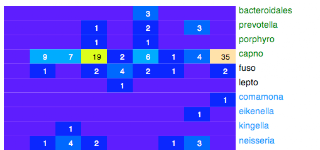### Window's Content ViewThis is in the "you learn something every day category." (As in, something obvious you should have noticed before). When displaying a view that will take up the whole window, I learned to grab a Custom View object from the palette in IB and dragged it to the window, then make it fill the window (and anchor it to the window for resizing). But, all you have to do really is to click on the blank window a second time. As with an NSTableView, you get different objects, shown using the Inspector. If you click once, you get "Window Identity." If you click twice, you get "View Identity." This is the contentView of the window! Just set the class name of that to be the one you define in code. No need for anything else.

### Mouse entering View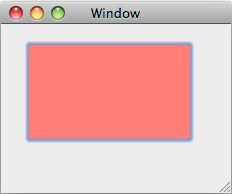On p. 274, Hillegass has an aside about how to pick up the event when a mouse enters and exits a view, e.g. to highlight the view, as we'll do here. Not the most exciting screenshot in the world, I know. I tried to get the mouse, but couldn't figure it out. The mouse tracking code is set up in "viewDidMoveToWindow," which I gather is called when that happens during launch. If we do that then we get events sent as calls to "mouseEntered_" and "mouseExited_." The highlighting part is handled in "drawRect_", with an important clean-up method below that. The other thing in this code is a demo of how to get additional colors besides the standard Apple ones, from the color list called "Crayons." Here is the code:

 `class MyView(NSView): def initWithFrame_(self, frame): self = super(MyView, self).initWithFrame_(frame) if self: return self def awakeFromNib(self): self.colorL = NSColorList.colorListNamed_('Crayons') self.isHighlighted = False def acceptsFirstResponder(self): return True def viewDidMoveToWindow(self): print 'viewDidMoveToWindow' print self.window().description() opts = NSTrackingMouseEnteredAndExited |\ NSTrackingActiveAlways |\ NSTrackingInVisibleRect ta = NSTrackingArea.alloc() ta.initWithRect_options_owner_userInfo_( self.bounds(), opts, self, None) self.addTrackingArea_(ta) def mouseEntered_(self, event): print 'mouseEntered_' self.isHighlighted = True self.setNeedsDisplay_(True) def mouseExited_(self, event): print 'mouseExited_' self.isHighlighted = False self.setNeedsDisplay_(True) def drawRect_(self, rect): salmon = self.colorL.colorWithKey_('Salmon') salmon.set() R = self.bounds() p = NSBezierPath.bezierPathWithRect_(R) p.fill() if self.window().firstResponder() == self: if NSGraphicsContext.currentContextDrawingToScreen(): if self.isHighlighted: NSGraphicsContext.saveGraphicsState() NSSetFocusRingStyle(NSFocusRingOnly) NSBezierPath.fillRect_(R) NSGraphicsContext.restoreGraphicsState() def resignFromFirstResponder_(self,sender): self.setKeyboardFocusRingNeedsDisplayInRect_(R) return YES`

## Monday, August 4, 2008

### Matrix Transposition

As part of my heat map project, I needed to transpose a matrix. I know I could use SciPy or hand-code a method, but I remembered an IAQ (infrequently asked question) from Peter Norvig where he gives this example:

 `m = [(1,2,3), (4,5,6)]zip(*m)# [(1, 4), (2, 5), (3, 6)]`

How does this work? Well, Norvig says that "f(*m) is like apply(f,m)," and I know that apply(f,m) feeds the elements of m to the function f, where in this case f is zip. If we do zip on two lists:

 `L = list('abcde')R = range(len(L))zip(L,R)# [('a', 0), ('b', 1), ('c', 2), ('d', 3), ('e', 4)]`

However, feeding the nested list directly to zip does not do what we want:

 `nestedL = [L,R]zip(nestedL)# [(['a', 'b', 'c', 'd', 'e'],), ([0, 1, 2, 3, 4],)]`

But this does:

 `apply(zip, nestedL)# [('a', 0), ('b', 1), ('c', 2), ('d', 3), ('e', 4)]`

So the next question is, how does f(*args) turn into apply(f,args)?
 `def f(*T): print type(T),Tf(nestedL)# < type 'tuple'> ([['a', 'b', 'c', 'd', 'e'], [0, 1, 2, 3, 4]],)`

T is a tuple containing nestedL as its first element. Here is where I lose the trail. It makes my head spin. But zip(*m) really is a neat trick to remember.

## Sunday, August 3, 2008

### Colors for heat maps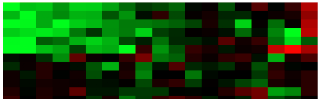If you are a scientist, you know about heat maps. A heat map is a method for representing a number from a distribution as a color. Typically the numbers are in matrices and the colors come from a distribution like the rainbow, or red <=> black <=> green. Heat maps are a great way of visualizing trends in data, and work particularly well with cluster analysis.

However, the choice of red and green for contrasting colors in classic work like Eisen et al. is unfortunate in view of the high incidence of defects in color vision. If I read the Wikipedia article correctly, the total frequency of phenotypes with negative impacts on color perception approaches 10% in males, although it would depend on the population. Frequency is much higher in males due to X-linkage of genes for some proteins involved in color vision, including receptors.

Another issue is the nature of the data. For microarray data, we want to know who is down, who is up and who is unchanged. Other data may be frequencies, ranging from 0 to 100%. For this case, so called "topological colors" are a good choice. They consist of shades of blue from light to dark, then green to yellow, and finally yellow into straw. I am not sure who invented this distribution.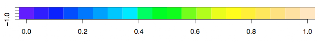R has standard functions for plotting heatmaps and generating colors (e.g. rainbow and topo.colors), which one calls with integer argument for the number of colors required from the distribution. I got interested in generating heat maps from Python using topological colors, so I wanted to know more about the distribution. I generated a vector of colors and examined their hex RGB values. The trends for most of the distribution are clear, but the last part was not. So I decided to plot the values. Shown here are the red, green and blue components of each topo.colors value with n = 1000, compared to the actual color generated in R.R is great at what it does easily, but I hate programming in it. I would rather use Perl, and that's saying a lot. Actually, it feels like a crazed hybrid between Perl and Lisp. I vowed not to spend another hour trying to figure out how to do something in R I can do in about 10 seconds in Python. Arghh... So the code I used to generate the plot is naturally, in Python:

 `import subprocess,os# part 1: write the R code, and call R L = ['n = 1000','f = "topo.colors.txt"','v = topo.colors(n)','write.table(v,f)']FH = open('.temp.Rcode.txt','w')FH.write('\n'.join(L))FH.close()cmd = 'R CMD BATCH ' + os.getcwd()cmd += '/' + '.temp.Rcode.txt'p = subprocess.Popen(cmd, shell=True)sts = os.waitpid(p.pid, 0)# part 2: basic processing of the R outputFH = open('topo.colors.txt','r')# first line is the name of the vectordata = FH.read().strip().split('\n')[1:]print len(data)data = [e.replace('"','') for e in data]# lose the '#' and the transparencydata = [e.split()[1:7] for e in data]# print data[:1]FH.close()# part 3# make dicts to convert hex values to 0..255L = range(256)L1 = [str(n) for n in L]# add leading '0' to first 10 entriesmodL = ['0' + e for e in L1[:10]]L1 = modL + L1[10:]L2 = [str(hex(i)[2:]) for i in L]# add leading '0' to first 16 hex entriesmodL = ['0' + e for e in L2[:16]]L2 = modL + L2[16:]L2 = [e.upper() for e in L2]D = dict(zip(L,L2))hD = dict(zip(L2,L))# part 4: separate red, green, blue# write data to diskL = [ [hD[e[:2]], hD[e[2:4]], hD[e[4:6]]] for e in data]L = [ [str(t), str(t), str(t)] for t in L]L = [(',').join(e) for e in L]FH = open('topo.colors.mod.txt','w')FH.write('\n'.join(L))FH.close()# part 5'''open R and paste:setwd('Desktop')v = read.csv('topo.colors.mod.txt',head=F)v[1:10,]x=1plot(x,type="n",xlim=c(0,1000),ylim=c(0,256))points(v[,1],col='red',cex=0.5,pch=16)points(v[,2],col='green',cex=0.5,pch=16)points(v[,3],col='blue',cex=0.5,pch=16)'''`

## Saturday, August 2, 2008

### Cocoa Selectors

I'm more than a little out of my depth here, but I'm going to speak up anyway.

Method names in Cocoa may be referred to loosely as "selectors." For example, on p. 34 Hillegass refers to insertObject:atIndex: as a selector, and Bob Ippolito does the same here. But a selector is really a special thing, not just a string, although it is connected to a string (the method name) by a lookup table. The system also stores type information about the function arguments. This latter aspect makes everything a little bit more complicated. (Much / all of what I'm saying here has been said before e.g. here. But I don't think that's a bad thing. Google isn't perfect yet).

According to the docs

the messaging routine has access to method implementations only through selectors, so it treats all methods with the same selector alike. It discovers the return type of a method, and the data types of its arguments, from the selector.

I don't think they mean "all methods," but "all methods in the same class."

The @selector() directive lets Objective-C source code refer to the compiled selector. Usually, we don't need to do this. The PyObjC bridge takes care of everything "under the hood."

Why does it matter? Well, we do need to refer to a selector any time we have to tell Cocoa what method to call. For example, in Objective-C we might do

 `[myButtonCell setAction:@selector(reapTheWind:)];`

or this in PyObjC:

 `oop = NSRunLoop.currentRunLoop() loop.performSelector_target_argument_order_modes_( "delayedResetFocus:", self, None, 9999, NSArray.arrayWithObject_(NSDefaultRunLoopMode) ) def delayedResetFocus_(self): self.myWindow.makeFirstResponder_(self.itemDescription) delayedResetFocus_ = objc.selector(delayedResetFocus_, signature = 'v@:')`

Recently in PyObjC we would reformulate this last part as

 ` @objc.signature('v@:') def delayedResetFocus_(self): self.myWindow.makeFirstResponder_(self.itemDescription)`

Or, we may want to run a process for the user that takes some time. Meanwhile, we could put up a sheet that is "modal" for the application, with a progress bar and a button giving the option to cancel. To do that, we will need to pass in a selector to the method we want to call when the sheet returns.

But the string passed to objc.signature is a shorthand for the types of the arguments and return value of the function, and it can get pretty complicated. Here is a little code to explore this. If you need a signature for a method, try to find a Cocoa method with the same arguments and / or return values and then ask it what its signature is.

 `import objcfrom Foundation import *sel = NSString.stringWithString_print sel.selector# stringWithString:print sel.signature# @12@0:4@8sel = NSString.lengthprint sel.selector# lengthprint sel.signature# I8@0:4class A(NSObject): @objc.signature('i8@0:') def anInt(self): return 2 @objc.signature('v@:') def notMuch(self): print 'Hi' a = A.alloc().init()print a.anInt()# 2a.notMuch()# Hisel = A.anIntprint sel.selector# anIntprint sel.signature# i8@0:sel = A.notMuchprint sel.selector# notMuchprint sel.signature# v@:`

### Cocoa Category in PyObjC

Continuing in the vein of "simple stuff about PyObjC," I thought I would show two examples of a Category. A Cocoa Category is what you do when you want to add new behavior to an existing class. That's contrasted with the situation that you want to change the behavior of an existing method in a class--- there you would use inheritance. This is explained in Apple docs. I found a nice example from Bob Ippolito (more detail here).

Here is a screenshot to show you the code with syntax coloring. (I've pasted in the commented Terminal output at various places).